# Flywheel

Flywheel turns 450 rev/min (RPM). Determine the magnitude of the normal acceleration of the flywheel point which are at a distance of 10 cm from the rotation axis.

Correct result:

g =  222.07 m/s2

#### Solution:We would be pleased if you find an error in the word problem, spelling mistakes, or inaccuracies and send it to us. Thank you!Tips to related online calculators
Do you want to convert velocity (speed) units?
Do you want to convert time units like minutes to seconds?

#### You need to know the following knowledge to solve this word math problem:

We encourage you to watch this tutorial video on this math problem:

## Next similar math problems:

• Propeller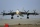The aircraft propeller rotates at an angular speed of 200 rad/s. A) What is the speed at the tip of the propeller if its distance from the axis of rotation is 1.5 m? B) What path does the aircraft travel during one revolution of the propeller at a speed o
• Circular motionMass point moves moves uniformly in a circle with radius r = 3.4 m angular velocity ω = 3.6 rad/s. Calculate the period, frequency, and the centripetal acceleration of this movement.
• BomberThe aircraft flies at an altitude of 4100 m above the ground at speed 777 km/h. At what horizontal distance from the point B should be release any body from the aircraft body to fall into point B? (g = 9.81 m/s2)
• Swing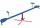A child weighing 12 kg is sitting on a swing at a distance of 130 cm from the axis of rotation. How far away from the axis of rotation (center) must sit down his mother weighs 57 kg if she wants to be swing in balance?
• GravitationFrom the top of the 80m high tower, the body is thrown horizontally with an initial speed of 15 m/s. At what time and at what distance from the foot of the tower does the body hit the horizontal surface of the Earth? (use g = 10 ms-2)
• Constant Angular Acceleration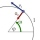The particle began to move from rest along a circle with a constant angular acceleration. After five cycles (n = 5), its angular velocity reached the value ω = 12 rad/s. Calculate the magnitude of the angular acceleration E of this motion and the time int
• Free fallThe free fall body has gone 10m in the last 0.5s. Find the body speed at the moment of impact.
• Inclined plane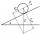On the inclined plane with an angle of inclination of 30 ° we will put body (fixed point) with mass 2 kg. Determine the acceleration of the body motion on an inclined plane.
• Shot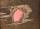Shot with a mass 43 g flying at 256 m/s penetrates into the wood to a depth 25 cm. What is the average force of resistance of wood?
• Motion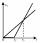The two bodies move in the same direction evenly in a straight line, at speeds of 5 cm/s and 10 cm/s. The movement of the first body started 2 seconds earlier than the movement of the second body, from a point located at a distance of 20 cm from the start
• Engine pulley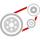The engine has a 1460 rev / min (RPM). Disc diameter is 350 mm. What will be the disc peripheral speed in RPM? Pulleys on the engine has diameter 80mm, on a disc has diameter 160mm.
• Two shipsThe distance from A to B is 300km. At 7 am started from A to B a ferry with speed higher by 20 km/h than a ship that leaves at 8 o'clock from B to A. Both met at 10h 24min. Determine how far they will meet from A and when they reach the destination.
• ParatrooperAfter the parachute is opened, the paratrooper drops to the ground at a constant speed of 2 m/s, with the sidewinding at a steady speed of 1.5 m/s. Find: a) the magnitude of its resulting velocity with respect to the ground, b) the distance of his land fr
• CarAt what horizontal distance reaches the car weight m = 753 kg speed v = 74 km/h when the car engine develops a tensile force F = 3061 N. (Neglect resistance of the environment.)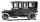Car started at 11:40 at speed 54 km/h from A and to B arrived at 12:20. Determine the distance A and B.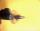Ball was fired at an angle of 35° at initial velocity 437 m/s. Determine the length of the litter. (g = 9.81 m/s2).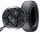Find the smallest coefficient of skid friction between the car tires and the road so that the car can drive at a 200 m radius at 108 km/h and does not skid.